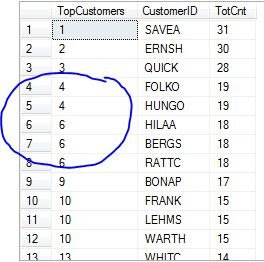## Friday, 10 August 2012

### RANK() VS DENSE_RANK()

The RANK()function in SQL Server returns the position of a value within the partition of a result set, with gaps in the ranking where there are ties.

The DENSE_RANK() function in SQL Server returns the position of a value within the partition of a result set, leaving no gaps in the ranking where there are ties.

Let us understand this difference with an example and then observe the results while using these two functions:

We will run two queries, one using RANK() and the other using DENSE_RANK() and observe the difference in the results. We will be using the ORDERS table of the NORTHWIND database to demonstrate the difference. The query will fetch the list of Customers ordered by the highest number of orders each has placed.

Using the RANK() function

SELECT RANK() OVER (ORDER BY TotCnt DESCAS TopCustomers, CustomerID, TotCnt

FROM (SELECT CustomerID, COUNT(*) AS TotCnt

FROM Orders Group BY CustomerID) AS Cust

OUTPUTAs shown in the results above, while using the RANK() function, if two or more rows tie for a rank, each tied rows receives the same rank, however with gaps in the ranking where there are ties. For example, Customers 'FOLKO' and 'HUNGO' have the same number of orders(i.e. 19), so they are both ranked 4. The Customers with the next highest order(HILAA, BERGS, RATTC) are ranked number 6 instead of 5, because there are two rows that are ranked higher at 4.

Using the DENSE_RANK() function

SELECT DENSE_RANK() OVER (ORDER BY TotCnt DESCAS TopCustomers, CustomerID, TotCnt

FROM (SELECT CustomerID, COUNT(*) AS TotCnt

FROM Orders Group BY CustomerID) AS Cust

OUTPUTAs shown in the results above, while using the DENSE_RANK() function, if two or more rows tie for a rank in the same partition, each tied rows receives the same rank, however leaving no gaps in the ranking where there are ties. Customers 'FOLKO' and 'HUNGO' have the same number of orders(i.e. 19), so they are both ranked 4. The Customers with the next highest order(HILAA, BERGS, RATTC) are ranked number 5. This is not the same as the RANK() function where the Customer with the next highest number of orders were ranked number 6.

Well I hope after seeing these example, you will understand the difference between the RANK() and DENSE_RANK() and will know where to use what.